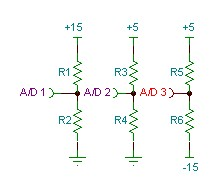## Saturday, March 5, 2016

### Interesting Circuit Problem

A while back I ran across a circuitry problem that may interest those with a little electronics knowledge. We were getting failures on a high percentage of new product, but not on all of them. The product was warning that its internal -15v supply was out of tolerance (and faulting). A manual check with a voltmeter showed the -15v supply was within the required +/-10% tolerance. Other internal supplies were monitored as well by the computer and they never exhibited this issue.

Below is a schematic of the components involved, what I found, and the interesting solution. What this schematic is showing is three resistor divider circuits that divide down the +15, +5v and the -15 volt supplies to feed the A/D converter inputs (there is some additional filtering and protection not shown).Scaling Circuitry
This A/D converter can resolve a range of 0v to +3v signal thus the need to divide down the voltages before conversion. Additionally the -15v rail voltage is 'translated' to a positive voltage by raising it with the +5V.  The code in the computer then reads the A/D converter and looks to see if the value read is out of +/-10% tolerance.

There is a problem though with the -15v divider circuit. Do you see it? The -15v circuit is dependent on the +5v voltage as well. So for instance if the +5v rail is 8% too high (within spec), and the -15v signal is 8% too low (also within spec), the resulting divided/translated voltage may be more than 10% out of whack (this is a highly technical term). The unit might then erroneously fault. I found a simple software change that put this issue to rest. We need to first read the +5 rail voltage. Then the -15v rail voltage is read and we subtract out the component of +5v rail voltage from the -15v reading. To do this requires some math and Kirchoff's voltage law.

VAD3 = V+5v - ( V+5v - V-15v) R5 /( R5 + R6

One note on the expression parameters,  V+5v  is the actual measured voltage of the +5V supply.   This is obtained by reading the VAD2 voltage first and calculating the +5V actual voltage. Next we have to solve the equation for the unknown voltage, V-15 :

V-15v = (V+5v - VAD3)(R5 + R6)/R5 + V+5v

Everything on the right hand side is known.  I implemented this equation in the firmware of the computer and it worked like a charm! I implemented it, but did not consider this solution to be optimal. In subsequent designs I made sure we did not use the +5v supply to translate the -15v supply voltage, but for this product this software solution had several benefits:

• Easy fix for units already in field. No recall.
• No waste for already manufactured inventory. We can use existing stuffed pcb's.
• We did not need to delay an already delayed product.
• No additional cost for the pcb NRE.
There were some other issues with this solution, scaling issues and floating point issues, but those are for another lab note.